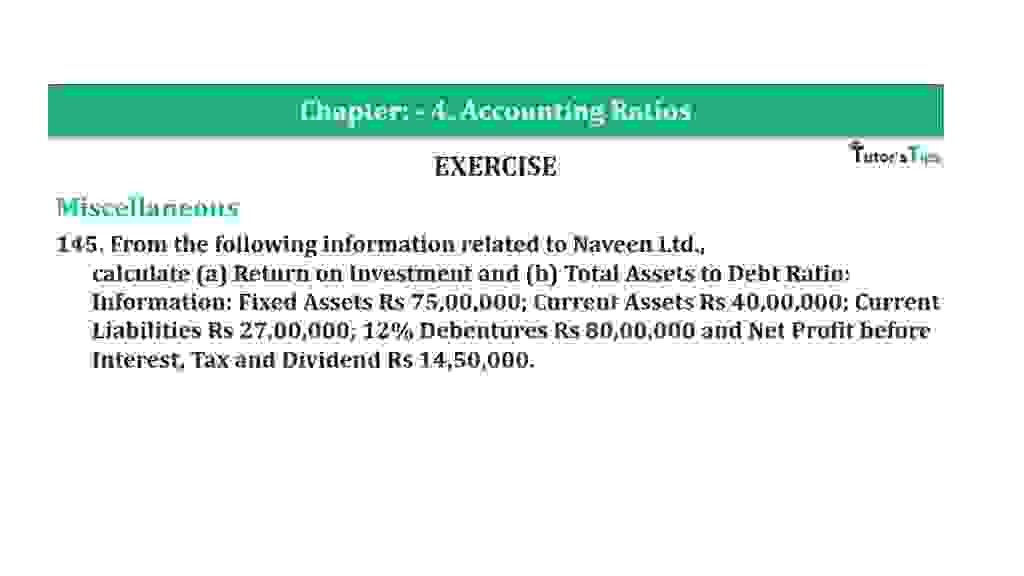# Question 145 Chapter 4 of +2-B – T.S. Grewal 12 ClassQuestion 145 Chapter 4 of +2-B

Miscellaneous

145. From the following information related to Naveen Ltd.,
calculate (a) Return on Investment and (b) Total Assets to Debt Ratio:
Information: Fixed Assets Rs 75,00,000; Current Assets Rs 40,00,000; Current Liabilities Rs 27,00,000; 12% Debentures Rs 80,00,000 and Net Profit before Interest, Tax and Dividend Rs 14,50,000.

### The solution of Question 145 Chapter 4 of +2-B: –

I

 Net Profit before Interest & Tax = Rs 14,50,000 Capital Employed = Fixed Assets + Current Assets – Current Liabilities = Rs 75,00,000 + Rs 40,00,000 – Rs 27,00,000 = Rs 88,00,000
 Return on Investment Ratio = Net Profit before Interest & Tax X 100 Capital Employed
 Return on Investment Ratio = Rs. 14,50,000 X 100 Rs 88,00,000 = 16.48%

II

 Total Assets = Fixed Assets + Current Assets = Rs 75,00,000 + Rs 40,00,000 = Rs 1,15,00,000 Long term Debts = Rs 80,00,000
 Total Assets to Debts Ratio = Total Assets Long term Debts
 = Rs. 1,15,00,000 Rs 80,00,000 = 1.44 : 1

Thanks, Please Like and share with your friends

Comment if you have any question.

Also, Check out the solved question of previous Chapters: –

### T.S. Grewal’s Double Entry Book Keeping (Vol. II: Accounting for Companies)T.S. Grewal’s Analysis of Financial Statements